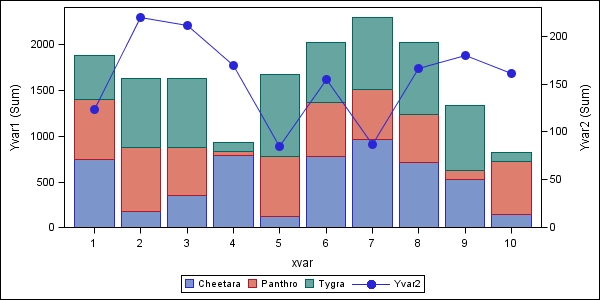## gbarline legend

hi experts,

is it possible for me to put the legend of the bar and the plot on a single frame without using an annotate dataset?

thanks!
milton
4 REPLIES 4

## gbarline legend

The following code creates a combined legend, containing both the bar & line, when I tried it in v9.2 and v9.2m3.

(I'm not 100% sure - is this what you're asking to do?)

data sample;
do cats='Cheetara','Panthro','Tygra';
do xvar=1 to 10;
yvar1=ranuni(123)*1000;
yvar2=ranuni(123)*100;
output;
end;
end;
run;

symbol1 v=dot i=join c=blue;

legend1; legend2;

legend1 frame label=('bar');
legend2 frame label=('plot');

proc gbarline data=sample;
bar xvar / discrete sumvar=yvar1
subgroup=cats legend=legend1;
plot / sumvar=yvar2 legend=legend2;
run;
quit;

## gbarline legend

In my effort to get one unified legend, that is, to get all of the variables in one line across the bottom, I modified Rob's code above. It's not an elegant solution. I am open to suggestions for a better way to do this.

data sample;
do cats='Cheetara','Panthro','Tygra';
do xvar=1 to 10;
yvar1=ranuni(123)*1000;
yvar2=ranuni(123)*100;
output;
end;
end;
run;

symbol1 v=dot i=join c=blue h=1 ;

options nocenter;
legend1; legend2;

legend1 noframe label=('' ) across=4 down=1
position=(bottom center outside)
origin=(10 pct ,1 pct)
value=('Cheetara' 'Panthro' 'Tygra' ' ')
shape=bar(.9,1)
mode=share;

legend2 noframe label=('' )  across=1 down=1
position=(bottom center outside)
origin=(51 pct ,1 pct)
value=('YVar')
shape=symbol(1.2,1)
mode=share;

axis2 origin=(, 20 pct);

proc gbarline data=sample;
bar xvar / discrete sumvar=yvar1
subgroup=cats maxis=axis2 legend=legend1;
plot / sumvar=yvar2 legend=legend2;
run;
quit;

## gbarline legend

Not sure if there's a more elegant way to get the legend you want, but you can make it look a little better by tweaking the 'shape' values a little, such as ...

symbol1 v=dot i=join c=blue h=1 ;

options nocenter;

legend1; legend2;

legend1 noframe label=('' ) across=4 down=1
position=(bottom center outside)
origin=(10 pct ,1 pct)
value=('Cheetara' 'Panthro' 'Tygra' ' ')
shape=bar(.15in,.15in)
mode=share;

legend2 noframe label=('' )  across=1 down=1
position=(bottom center outside)
origin=(51 pct ,1 pct)
value=('YVar')
shape=symbol(7,1.5)
mode=share;

axis2 origin=(, 20 pct);

title;

proc gbarline data=sample;
bar xvar / discrete sumvar=yvar1
subgroup=cats maxis=axis2 legend=legend1;
plot / sumvar=yvar2 legend=legend2;
run;
quit;Jay54
Meteorite | Level 14

## Re: gbarline legend

If you are using SAS 9.2 or later, you can use GTL to create this graph.  GTL Code and output attached.

If you are using SAS 9.3, you can use the simpler SGPLOT procedures too.

Output:V9.2 GTL code:

```/*--Summarize the data for the series overlay--*/
proc means data=sample nway;
class  xvar;
output out= sampleSum sum(yvar2) = Yvar;
run;
/*--Merge summarized column with original data--*/
data sample2;
merge sample (rename=(xvar=xvar1)) samplesum(keep=xvar Yvar);
run;

/*--Bar line template--*/
proc template;
define statgraph barlinelegend;
begingraph;
layout overlay / yaxisopts=(offsetmin=0 label='Yvar1 (Sum)' linearopts=(viewmin=0))
y2axisopts=(offsetmin=0 label='Yvar2 (Sum)' linearopts=(viewmin=0));
barchart x=xvar1 y=yvar1 / group=cats name='bar';
seriesplot x=xvar y=yvar / name='line' yaxis=y2 legendlabel='Yvar2'
display=(markers) markerattrs=graphdata1(symbol=circlefilled size=11)
lineattrs=graphdata1;
discretelegend 'bar' 'line';
endlayout;
endgraph;
end;
run;
/*--Render the graph--*/
ods listing;
ods graphics / reset width=6in height=3in imagename='BarLineLegendGTL';
proc sgrender data=sample2 template=barlinelegend;
label xvar1='xvar';
run;
```
Discussion stats
• 4 replies
• 1394 views
• 0 likes
• 4 in conversation